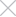We track sessions with cookies For what?Select a languageSeptember 19, 2022#tech

# Part 2. How do navigation apps take the shortest route?

Continuing the theme of the last article, we will talk about Dijkstra's Algorithm. What is it for and what problems it helps to solve.

Everyone remember the graph we used to represent bus routes from point A to point B?

But suppose each segment (edge) is associated with a travel duration. And then it turns out that there is a faster path. So, the problem we were solving wouldn't really fit the situation if we had our own car and wanted to get from home to work by car.

At the end of last week's part, I showed a graph. The same graph, but it has added weights, namely the length of roads we can take to get to our jobs. Let's look at that graph again.

Such graphs are called weighted graphs. The terminology of a weighted graph remains the same. But their key feature is that we can use as weights any values, whether it is time spent to get from one node to another, or money spent to get to the destination.

The breadth search, which we dealt with in the previous chapter, does not know how to handle weighted graphs. It only looks for the shortest path (the shortest number of segments, edges, that we can traverse to get to the destination).

Below we will change our graph a bit, for a better example.

Here, this will be good enough to break down the main points of Dijkstra's Algorithm on this graph.

The Dijkstra Algorithm consists of four basic steps:

• Find the node with the lowest cost (weight);

• Update the cost of that node's neighbors;

• Repeat until this is done for all nodes in the graph;

• Calculate the final path.

Let's try to apply this algorithm.

Before we start, let's assume that the weight of nodes that we can't reach in 1 move is equal to infinity.

Let's create a little table, in which we will enter data as we use the algorithm. Initially it will look like this:

Step 1. Find the node with the lowest cost. In this case the shortest road we can take is from A to D.

Step 2. Calculate how long it will take to get to all its neighbors.

The cost of node E is entered into the table, and node D is assigned as its parent. But we also found a shorter route to node C. We can get to it with the lowest cost, so we update the cost of it and its parent.

Again step 1. The next lowest cost is node C.

Again step 2. Update the values of all its neighbors

Now, the values of nodes E and B have been updated. This means that it is faster to get to node E through the edge coming from node C. And we finally found our first route to point B.

Now its cost is only 8. But the work of our algorithm is not over at this point. Let us try to repeat the steps.

Step 1 again. The next most expensive node is node E.

Step 2 again. Update the values of all its neighbors

So we found a shorter path to node B. Its cost will be - 6. If we move through the edge coming from node E.

There is no point in continuing our algorithm, because node B is finite.

To see what our shortest route looks like, we can build a chain of all parents coming from node B. So our route will look like this: A - D - C - E - B.

Now let's try to write the code of such an algorithm:

const graph = {

'A': {

neighbors: {

'C': 5,

'D': 2

},

parent: null,

cost: null

},

'D': {

neighbors: {

'E': 4,

'C': 2

},

parent: 'A',

cost: 2

},

'C': {

neighbors: {

'E': 1,

'B': 3

},

parent: 'A',

cost: 5

},

'E': {

neighbors: {

'B': 1

},

parent: null,

cost: Infinity

},

'B': {

neighbors: {},

parent: null,

cost: Infinity

}

}

const processed = [];

const find_lowest_cost_node = (graph) => {

let result, value = Infinity

Object.keys(graph).forEach((node) => {

if (!processed.includes(node) && typeof graph[node].cost === 'number') {

if (graph[node].cost < value) {

value = graph[node].cost

result = node

}

}

})

return result

}

const search_quick_way = (graph, endpoint) => {

let way = [endpoint]

while (graph[endpoint].parent) {

way.push(graph[endpoint].parent)

endpoint = graph[endpoint].parent

}

return way.reverse().join(' - ')

}

const search = (graph) => {

let node = find_lowest_cost_node(graph)

while (node) {

let cost = graph[node].cost

let neighbors = graph[node].neighbors

for (let n in neighbors) {

const new_cost = cost + neighbors[n]

if (graph[n].cost > new_cost) {

graph[n].cost = new_cost

graph[n].parent = node

}

}

processed.push(node)

node = find_lowest_cost_node(graph)

}

return graph

}

That's it, we've implemented the Dijkstra Algorithm.

You may ask, but how do navigation applications take into account traffic jams?    It's simple. If there is a traffic jam on the street, we can increase the weights of our edges. For example, we can add some coefficients that will change our weights, and they will become much larger than others.

Thank you all for your attention!